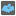• # Not working on opacity 6736

## Question related to missionGhosts Age

It works on every example but it doesn't work on 6736! Actually, temp_opacity and opacity are not getting equal in this example!

```fibonacci_list = []
```

def checkio(opacity): """ This returns the age of a ghost based on its opacity.

```>>> chechio(1000)
0
>>> checkio(9997)
3
"""
temp_opacity = 10000
age = 0
dif = 10000 - opacity
fibonacci()

for i in range(1, dif+1):
if temp_opacity == opacity:
age = i - 1
break
if is_fibonacci(i):
temp_opacity = temp_opacity - i
elif not is_fibonacci(i):
temp_opacity += 1

return age
```

def fibonacci(): a, b = 0, 1 global fibonaccilist while b <= 5000: fibonaccilist.append(b) a, b = b, a+b

def isfibonacci(number): return True if number in fibonaccilist else False9